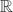Hostname: page-component-7d684dbfc8-hsbzg Total loading time: 0 Render date: 2023-09-27T16:48:58.926Z Has data issue: false Feature Flags: { "corePageComponentGetUserInfoFromSharedSession": true, "coreDisableEcommerce": false, "coreDisableSocialShare": false, "coreDisableEcommerceForArticlePurchase": false, "coreDisableEcommerceForBookPurchase": false, "coreDisableEcommerceForElementPurchase": false, "coreUseNewShare": true, "useRatesEcommerce": true } hasContentIssue false

# Hölder differentiability of self-conformal devil's staircases

Published online by Cambridge University Press:  09 January 2014

## Abstract

In this paper we consider the probability distribution function of a Gibbs measure supported on a self-conformal set given by an iterated function system (devil's staircase) applied to a compact subset of${\mathbb R}$. We use thermodynamic multifractal formalism to calculate the Hausdorff dimension of the sets Sα0, Sα and Sα, the set of points at which this function has, respectively, Hölder derivative 0, ∞ or no derivative in the general sense. This extends recent work by Darst, Dekking, Falconer, Kesseböhmer and Stratmann, and Yao, Zhang and Li by considering arbitrary such Gibbs measures given by a potential function independent of the geometric potential.

Type
Research Article

## Access options

Get access to the full version of this content by using one of the access options below. (Log in options will check for institutional or personal access. Content may require purchase if you do not have access.)

## References

#### REFERENCES

Bowen, R.Equilibrium states and the ergodic theory of Anosov diffeomorphisms, Lecture Notes in Math. vol. 470 (Springer-Verlag, Berlin, revised edition, 2008). With a preface by David Ruelle, Edited by Jean-René Chazottes.Google Scholar
Darst, R.The Hausdorff dimension of the nondifferentiability set of the Cantor function is [ln(2)/ln(3)]2. Proc. Amer. Math. Soc. 119 (1) (1993), 105108.Google Scholar
Darst, R.Hausdorff dimension of sets of non-differentiability points of Cantor functions. Math. Proc. Camb. Phil. Soc. 117 (1) (1995), 185191.CrossRefGoogle Scholar
Falconer, K.Techniques in Fractal Geometry. (John Wiley & Sons Ltd., Chichester, 1997).Google Scholar
Falconer, K. J.One-sided multifractal analysis and points of non-differentiability of devil's staircases. Math. Proc. Camb. Phil. Soc. 136 (1) (2004), 167174.CrossRefGoogle Scholar
Kesseböhmer, M. and Stratmann, B. O.Hölder-differentiability of Gibbs distribution functions. Math. Proc. Camb. Phil. Soc. 147 (2) (2009), 489503.CrossRefGoogle Scholar
Li, W.Non-differentiability points of Cantor functions. Math. Nachr. 280 (1–2) (2007), 140151.CrossRefGoogle Scholar
Morris, J.The Hausdorff dimension of the nondifferentiability set of a nonsymmetric Cantor function. Rocky Mountain J. Math. 32 (1) (2002), 357370.CrossRefGoogle Scholar
Pesin, Y. and Weiss, H.The multifractal analysis of Gibbs measures: motivation, mathematical foundation, and examples. Chaos 7 (1) (1997), 89106.CrossRefGoogle ScholarPubMed
Pesin, Y. B.Dimension theory in dynamical systems. Chicago Lectures in Mathematics. (University of Chicago Press, Chicago, IL, 1997). Contemporary views and applications.CrossRefGoogle Scholar
Ruelle, D.Thermodynamic formalism. Cambridge Mathematical Library (Cambridge University Press, Cambridge, second edition, 2004). The mathematical structures of equilibrium statistical mechanics.CrossRefGoogle Scholar
Yao, Y., Zhang, Y. and Li, W.Dimensions of non-differentiability points of Cantor functions. Studia Math. 195 (2) (2009), 113125.CrossRefGoogle Scholar